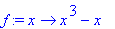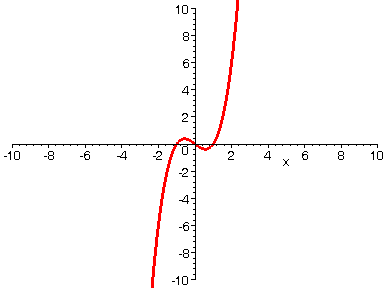HORIZONTAL STRETCH

If you take a function y = f(x) and replace it by y = F(k*x) where 0 < k < 1, then the net result is that the graph of f(x) is stretched horizontally away from the vertical axis. Below we start with a polynomial function of the form y = f(k*x), and the animation shows the effect on the graph as we let k decrease from 1 to 1/20. By the way, this effect looks similar to that of a vertical compression. How can we tell the difference?

> with(plots):

> f:=x->x^3-1*x;> A := seq(plot(f((1/i)*x), x=-10..10, numpoints=500, thickness=3), i=1..20):

> display(A, insequence=true, view=[-10..10, -10..10]);>